# Test: Prime Numbers- 2

## 20 Questions MCQ Test Quantitative Reasoning for GMAT | Test: Prime Numbers- 2

Description
Attempt Test: Prime Numbers- 2 | 20 questions in 40 minutes | Mock test for GMAT preparation | Free important questions MCQ to study Quantitative Reasoning for GMAT for GMAT Exam | Download free PDF with solutions
QUESTION: 1

### A positive integer n has 3 prime factors. Is n odd? (1) The total number of factors of n is odd. (2) The prime factors of n when arranged in ascending order form an evenly spaced set.

Solution:

Step 1 & 2: Understand Question and Draw Inference

Given:

• Positive integer n
• n has 3 prime factors
• therefore we can write,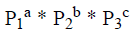where a, b, c ≥ 1.

To find: is n odd?
To determine the even-odd nature of n, we need to see if 2 is one of the prime
factors (P1 or P2 or P3 ) of n

Step 3 : Analyze Statement 1 independent

1. The total number of factors of n is odd
The statement tells us that n is a perfect square, but it does not tell us if 2 is one of the prime factors of n.
Hence, statement-1 is insufficient to answer the question.

Step 4 : Analyze Statement 2 independent

2. The prime factors of n when arranged in ascending order form an evenly spaced set.
As P1 , P2 , P3 form an evenly spaced set, we can write {P1 , P2 , P3} = { P1,P1 +d, P1 +2d} assuming P1 < P2 < P3
So, P + P = 2P i.e. P + P is even.
This would be possible only if P1 and P3 both are odd. P1 and P3 both can’t be even as there is only one even prime number possible.
As P2 lies between P1 and P3, P2 also has to be odd as there is no even prime number which lies between two odd prime numbers.
Hence none of P1 , P2, or P3 is 2. Thus n is definitely odd.
So, statement-2 is sufficient to answer the question.

Step 5: Analyze Both Statements Together (if needed)

Since, we have a unique answer from step 4, this step is not required.

QUESTION: 2

### The product of the largest two factors of a positive integer n is 16875. What is the difference between the largest positive integer that divides √n and the smallest odd factor greater than 1 of √n?

Solution:

Given:

• n is an integer > 0
• Let a and b respectively be the two largest factors of n
• a * b = 16875

To Find: Difference between the largest positive factor that divides √n and the smallest odd factor of √n greater than 1.

• Largest positive factor that divides √n will be √n itself
• Smallest odd factor of √n greater than 1) = (smallest odd prime factor of √n)

Approach:

1. To find the factors of √n, we first need to find the value of √n. For finding the value of √n, we need to find the value of n.
2. We are given information about the product of the largest two factors of n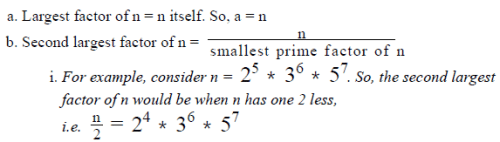c. For finding the smallest prime factor of n, we need to find the smallest prime factor of 16875.

• This is because the prime factors of 16875 will only be the prime factors of n, as 16875 is the product of n with one of the factors of n.

Working out: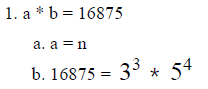c. As the smallest prime factor of 16875 is 3, the second largest factor of n = n/3 =b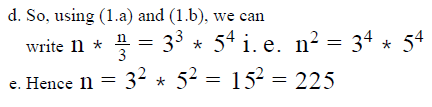2. So, √n = 15
3. Largest positive factor that divides √n = √n = 15
4. Smallest odd factor of √n greater than 1 = smallest odd prime factor of √n = 3
5. So, Largest positive factor that divides √n - Smallest odd factor of √n greater than 1 = 15 – 3 = 12

QUESTION: 3

### For a positive integer n, the function @n represents the product of all even numbers that lie between n and 2n, exclusive. For example, @6 = 8*10. If k is a positive integer and the greates  prime factor of @k is 17, which of the following cannot be the value of k? I. 9 II. 18 III. 32

Solution:

Given:

• A positive integer k
• @k = Product of all even integers between k and 2k, exclusive
• The greatest Prime factor of @k = 17

To Find: Which of the given 3 values doesn’t satisfy the given information about k

Approach:

1. We will find out the expression for @k for each of the 3 given values
2. We’ll determine the prime factors of @k to check if 17 is the greatest

prime factor:
a. If the answer is YES, then that value of k is possible
b. If the answer is NO, then that value of k is not possible

Working out: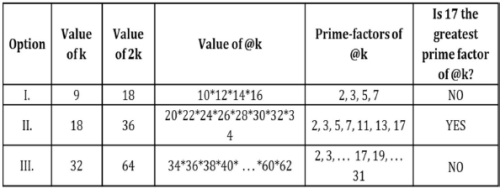Looking at the answer choices, we see that the correct answer is Option D From the table, it’s clear that out of the 3 given values, Options I and III (9 and 32) are not possible (Remember that the question is asking which values are not possible)

QUESTION: 4

For any integer P greater than 1, P! denotes the product of all the integers from 1 to P, inclusive. The value of 11! is closest to
which of the following powers of 10?

Solution:

Given:

• For any positive integer P, P! = P(P-1)(P-2) . . . * 3* 2 * 1
• Since 11 is a positive integer, 11! = 11*10*9*8*7*6*5*4*3*2*1

To Find: Which power of 10 is 11! The closest to?

Approach:

1. To answer this question, we’ll use the principle of Estimation and Rounding
2. In the first step, we’ll group the terms in the expression for 11! such that the product of 2 or more terms in a group is close to a multiple of 10.
3. Then, we’ll round the products obtained in the first step to multiples of 10 and estimate the value of 11!

Working out: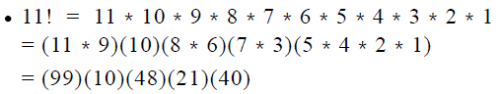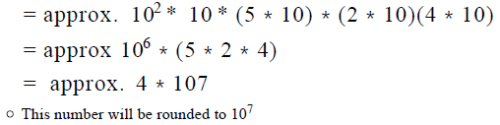Looking at the answer choices, we see that the correct answer is Option B

QUESTION: 5

Does the positive integer M have greater than 4 factors?
(1) M/8 is an integer
(2) M/20 is an integer

Solution:

Step 1 & 2: Understand Question and Draw Inference

Given: Positive integer M
To find:

• Is Total number of factors of M > 4?
• Total number of factors = (m+1)(n+1)(p+1) . . . .

Step 3 : Analyze Statement 1 independent

• M/8 is an integer
• So, M is divisible by 8
• 8 = 23
• So, M contains 2 or a higher power of 2
• We can write the prime-factorized form of M as:

M = 23+a *P2b * P3c . . . where a, b, c . . . are non-negative integers

• So, total number of factors of M = (3 + a + 1) (b + 1) (c + 1) ...
• If a = b = c ... = 0, total number of factors of M = (3 + 1) = 4.
• So the answer to the question (Is total number of factors greater than 4?) is NO in this case
• For all other values of a, b, c . . . , the answer to the question is YES.

Thus, Statement 1 is not sufficient to arrive at a definite answer to the question.

Step 4 : Analyze Statement 2 independent

• M/20 is an integer
• So, M is divisible by 20
• 20 = 22 x 5
• So, M contains 22 (or a higher power of 2) and 5 (or a higher power of 5)
• We can write the prime-factorized form of M as: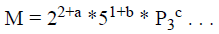where a, b, c . . . are non-negative integers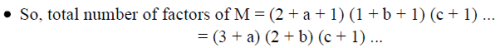• If a = b = c ... = 0, total number of factors of M = (3)(2) = 6.
• So the answer to the question is YES in this case
• For all other values of a, b, c . . . also, the answer to the question is YES.

Thus, Statement 2 is sufficient to provide us a definite answer to the question.

Step 5: Analyze Both Statements Together (if needed)

Since we’ve already arrived at a unique answer in Step 4, this step is not required

QUESTION: 6

p is a positive integer greater than 100. Is p divisible by 36?
(1) There are 8 positive integers, including 1 and p, which divide p.
(2) The highest positive integer less than or equal to 100 that divides p is 75.

Solution:

Step 1 & 2: Understand Question and Draw Inference

The question tells us about a positive integer p greater than 100. It asks us to find if p is divisible by 36
Let’s first analyze the information given in the question statement.
36 = 22 * 32 . For p to be divisible by 36, p should have at least 2 and 3 in it.
Also, since 36 has (2+1) (2+1) = 3 *3 = 9 factors, p should have a minimum of 9 factors.
With the above understanding, let’s see the information provided in the statements.

Step 3 : Analyze Statement 1 independent

1. There are 8 positive integers, including 1 and p, which divide p Statement-1 tells us that p has a total of 8 number of factors. We know that for p to be divisible by 36, it should have a minimum of 9 factors. As p has less than 9 factors, p is definitely not divisible by 36.
Hence statement-1 is sufficient to answer the question.

Step 4 : Analyze Statement 2 independent

2. The highest positive integer less than or equal to 100 that divides p is 75.
Statement-2 tells us that 75 is the highest integer less than equal to 100 which divides p.
75 = 52* 3. Had p been divisible by 36, p should also have contained at least 2 and 3 .

So, p would have at least 2 , 3 and 5 in it. With this combination of the prime factors and their powers, the highest integer less than equal to 100 which divides p should have been 5 * 2 = 100. As 75 is the highest integer less than equal to 100 which divides p, we can say that p does not contain 22 . So p is definitely not divisible by 36.
Hence statement-2 is sufficient to answer the question.

Step 5: Analyze Both Statements Together (if needed)

Since we have a unique answer from steps 3 and 4, this step is not required.

QUESTION: 7

Can the total number of integers that divide x be expressed in the form of 2k + 1, where k is a positive integer?
(1) √12x is an integer
(2) The product of √x and √y is an integer, where the total number of factors of y/3 is odd.

Solution:

Step 1 & 2: Understand Question and Draw Inference

• We know that a number in the form of 2k + 1 leaves a remainder of 1 when divided by 2, (that is, a number that leaves a remainder of 1 when divided by 2) is odd.
• So, we are asked to find if the number of factors of x is odd.

To Find: Is the number of factors of x odd?

• We know that if a number has odd number of factors, it has to be a perfect square. So, we are (indirectly) asked to find if x is a perfect square?

Step 3 : Analyze Statement 1 independent

1. √12 x is an integer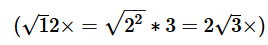is an integer. For √3x to be an integer,
x should contain an odd power of 3.
Now, if 3 occurs odd number of times in x, x can’t be a perfect square.
Hence statement-1 is sufficient to answer the question.

Step 4 : Analyze Statement 2 independent

2. The product of √x and √y is an integer, where the total number of factors of y/3 is odd.
Statement-2 tells us that the total number of factors of y/3 is odd i.e. y/3  is a perfect square. Let’s assume  y/3 = 3z2. Since z2 is always non-negative, y will also be a non-negative integer

So, we know that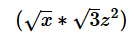is an integer i.e. z√3x is an integer.

For √3x to be an integer, x should contain an odd power of 3. If 3 occurs odd
number of times in x, x can’t be a perfect square
Hence statement-2 is sufficient to answer the question.

Step 5: Analyze Both Statements Together (if needed)

Since we have a unique answer from steps 3 and 4, this step is not required.

QUESTION: 8

If m has the smallest prime number as its only prime factor, is  ∛m an integer?
(1) m2 is divisible by 32
(2) √m is divisible by 4

Solution:

Step 1 & 2: Understand Question and Draw Inference

Given:

• Positive integer m
• The only prime factor of m = the smallest prime number
• The smallest prime number is 2
• So, m = 2n , where n is a positive integer

To find: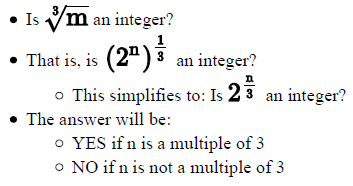So, in order to answer the question, we need to find if n is a multiple of 3 or not

Step 3 : Analyze Statement 1 independent

• m2 is divisible by 32
• m2 = 22n
• 32 = 25
• Since m2 is divisible by 32, m2 must be greater than or equal to 32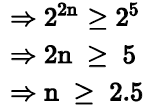• Since n is an integer, the minimum possible value of n is 3.
• So possible values of n = {3, 4, 5, 6 . . . }
• Thus n may or may not be a multiple of 3

Step 4 : Analyze Statement 2 independent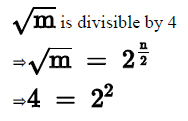Since √m is divisible by 4,  √m  must be greater than or equal to 4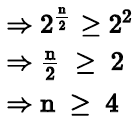• So possible values of n = {4, 5, 6 . . . }
• Thus n may or may not be a multiple of 3

Statement 2 alone is not sufficient to answer the question.

Step 5: Analyze Both Statements Together (if needed)

• From Statement 1: n = {3, 4, 5, 6 . . . }
• From Statement 2: n = {4, 5, 6 . . . }
• By combining both statements: n = {4, 5, 6 . . . }

We’ve still not been able to determine if n is a multiple of 3.
So, even the two statements together are not sufficient to answer the question.

QUESTION: 9

For any integer P greater than 1, P! denotes the product of all the integers from 1 to P, inclusive. In how many ways can 9! be
expressed as a product of 2 positive integers?

Solution:

Given:

P > 1
P! = 1 x 2 x 3…x P

To Find
# of ways
in which 9! can be written
as a x b , where a, b are integers > 0

Approach:

1. Note the nature of numbers a,b in the product . a x b.
a,b are both factors of 9!.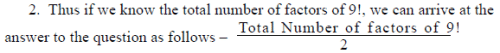Let’s take a simple example to understand the above application. Let’s take the number 6.
Its factors are – 1, 2, 3, 6 - i.e. 4 factors
It can be expressed as product of two numbers as follows
1 x 6
2 x 3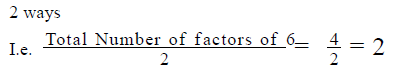Working out: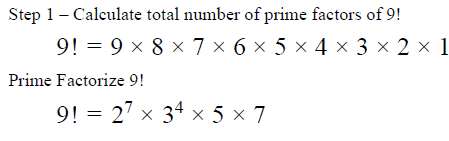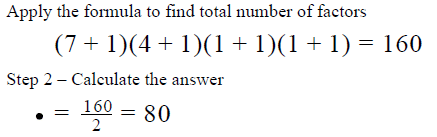9! can be expressed as a product of two positive numbers in 80 ways. Choice D is the correct answer.

QUESTION: 10

What percentage of odd prime numbers lying between 1 and 30 divide 7,700 completely?

Solution:

Given: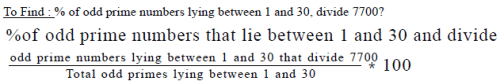Approach: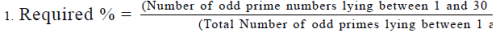2. For finding the prime numbers that divide 7700, we need to find the prime factors of 7700, which will tell us the odd prime numbers that
divide 7700

3. Then we will find the number of odd prime numbers lying between 1 and 30.

4. Using (1) and (2), we can find the percentage of odd prime numbers lying between 1 and 30, that divide 7700

Working out: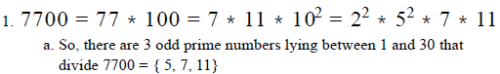2. Number of odd prime numbers lying between 1 and 30 = {3, 5, 7, 11, 13, 17, 19, 23, 29} = 9 odd prime numbers.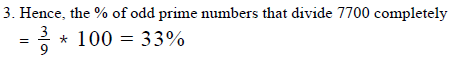QUESTION: 11

If the dimensions of a rectangle (in inches) is equal to a prime number that lies between 55 and 65, exclusive, which of the
following statements must be true?
I. There is only one other rectangle that has integral dimensions and the same area as the given rectangle
II. There is only one other rectangle that has integral dimensions and the same perimeter as the given rectangle
III. The area of the given rectangle is 3599 square inches.

Solution:

Given:

• Length of the rectangle (inches) = {59, 61}
• Breadth of the rectangle (inches) = {59, 61}
• Note that we’re not given that Length and Breadth are distinct integers.

So, it would be wrong to assume so.

To Find: Which of the 3 given statements must be true?

Approach:

1. We’ll evaluate the 3 statements one by one. They will qualify as must be true statements only if no exception is found to those statements.

Working out:

•  Evaluating Statement I
• ​There is only one other rectangle that has integral dimensions and the same area as the given rectangle
• This statement talks about the area of the given rectangle. So, let us first see what are the different possible values for the area of the given rectangle. We find that 3 values of area are possible:
• 59*59
• 59*61
• 61*61
• Let’s evaluate the first possibility, where the area of the given rectangle is 59*59

In how many ways can 59*59 be expressed as a product of 2 integers?

• Way 1 - (59)*(59)
• Way 2 - (1)*(59*59)

• This means, only 2 rectangles are possible whose area is 59*59:
• A rectangle each of whose side is 59 inches
• This is the given rectangle
• A rectangle whose one side is 1 inch and the other is 59*59 inches
• Thus, apart from the given rectangle, there is only one other rectangle that can have the same area as the given rectangle
• We can do a similar analysis for the other 2 possible values of the area (59*61 and 61*61). We’ll get the same result – that there is only one other rectangle that can have the same area as the given rectangle
• The reason why the other 2 values will also yield the same result is that these 2 values of the area too are a product of prime numbers, like the first possibility was. So, the analysis and the conclusion will be similar.
• Thus, Statement 1 holds true for each of the 3 possible values of the area of the given rectangle. Therefore, this statement is a must be true statement.

• Evaluating Statement II
• There is only one other rectangle that has integral dimensions and the same perimeter as the given rectangle
• This statement talks about the perimeter of the given rectangle. So, let’s first evaluate what the perimeter of the given rectangle will be. We find that 3 values are possible for the perimeter:
• 2(59+59)
• 2(59+61)
• 2(61+61)
• Let’s evaluate the first possible value of the perimeter, 2(59+59) and see
how many different rectangles can have this perimeter
• All the following pairs of length and breadth lead to the same perimeter:
• (59 – 1, 59 + 1), that is, (58, 60)
• (59 – 2, 59 + 2), that is, (57, 61)
• (59 – 3, 59 + 3), that is, (56, 62)
• And so on . . .
• Thus, we see that there are more than one rectangles that can have the same perimeter as the given rectangle if the perimeter of the given rectangle is 2(59+59)
• Since Statement II has proved untrue for one possible value of the Perimeter, it is definitely not a must be true statement.
• Note: You may have done your analysis with one of the 2 other possible values of the perimeter. That’s perfectly okay. The line of reasoning will be the same and will lead to the same conclusion as above.

• Evaluating Statement III
• The area of the given rectangle is 3599 square inches.

• As discussed in Statement I, 3 values of Area of the given rectangle are possible:
• 59*59
• 59*61
• 61*61
• Therefore, we cannot say definitely what the area of the given rectangle is. We can only say that the area can be 59*59 or 59*61 or 61*61 square inches
• So, Statement III is not true.

Looking at the answer choices, we see that the correct answer is Option A

QUESTION: 12

If k is a positive integer and 6k3 is divisible by 2500, then which of the following statements must be true?
I. k is divisible by 50
II. 20 is a factor of k
III. k5 is divisible by 64

Solution:

Given:

• Positive integer k (so, k > 0)
• 6k is divisible by 2500

To Find: Which of the 3 statements must be true?

Approach:

1. Since the 3 statements talk about k and the higher powers of k, we will first draw inferences about k from the given information.
2. Then, we’ll evaluate the 3 statements one by one. They will qualify as must be true statements only if no exception is found to those statements.

Working out: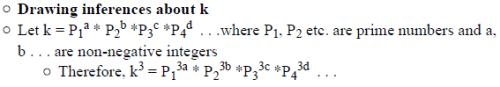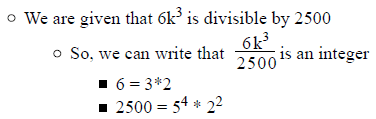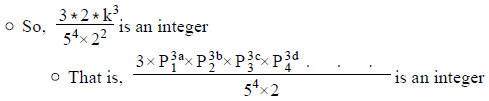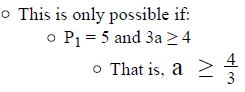Since a is an integer, minimum possible value of a = 2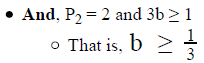Since b is an integer, minimum possible value of b = 1

Therefore, k = 2*52* P3c *P4d . . .

• Evaluating Statement I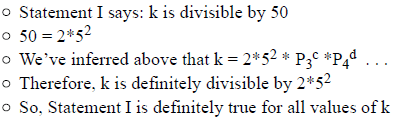• Evaluating Statement II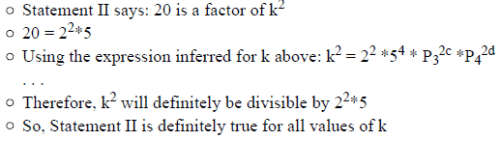• Evaluating Statement III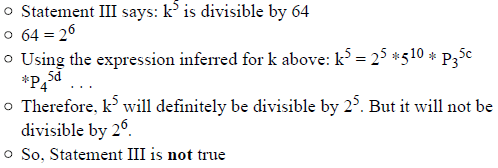Looking at the answer choices, we see that the correct answer is Option D

QUESTION: 13

A class of students appeared in two different tests of quantitative ability both of which were scored out of 100. The scores of each
student in each test could be expressed as a product of two distinct prime numbers. If a student’s scores in the two tests did not have
any common prime factors, what is the maximum possible difference between his two scores?

Solution:

Given:

• Students of a class appeared in 2 tests score out of 100
• Scores of each student = p * q, where p, q are two distinct prime numbers
• A student’s score in both the tests did not have any common prime factor

To Find: Maximum possible difference between the scores of a student in two tests

• Highest possible score – Lowest possible score

Approach:

1. We need to find the highest possible and the lowest possible score
2. Lowest possible score
• As the score = p * q, where p and q are prime numbers, the lowest possible score would be when p and q are the lowest possible prime numbers.
3. Highest possible score
• As the maximum score that a student can score is 100, for calculating the highest possible score, we will start checking the numbers backwards from 100 to see, which is the highest number that can be expressed as a product of 2 distinct prime numbers,
• However, we need to keep in mind that the prime factors of the highest possible score are not common to the prime factors of the lowest possible score.

Working out:

1. Lowest Possible score
• Lowest possible score = p * q, where p and q are the smallest prime factors possible.
• So, (p, q) = (2, 3).
• Thus, the lowest possible score = 2 * 3 = 6
2. Highest possible score
• Let’s start the counting backwards from 100. Note that none of even scores are possible as the highest possible scores because we have already taken 2 to be the prime factor of the lowest possible score. So, we just need to check the odd scores
• 99 = 3 * 33. Not possible to express it as a product of 2 distinct primes
• 97 = Prime, cannot be expressed as product of 2 distinct primes. Not possible
• 95 = 19 * 5. Possible to express it as a product of 2 distinct primes. Also none of the prime factors of 19 and 5 are common to the prime factors of the lowest possible score (6). So, it is a valid score.
• Thus, the highest possible score = 95
3. Hence, the maximum difference between the scores = 95 – 6 = 89

QUESTION: 14

A positive integer n has more than 1 distinct prime factor. Is the product of the prime factors of n less than n?
(1) The number of distinct factors of n, including n, is odd.
(2) n can be expressed as a multiple of 4.

Solution:

Step 1 & 2: Understand Question and Draw Inference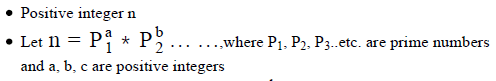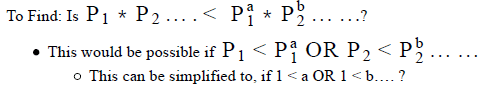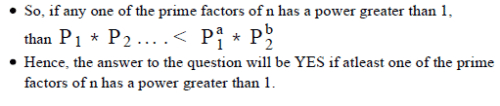Step 3 : Analyze Statement 1 independent

1. The number of distinct factors of n, including n, is odd.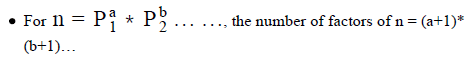• We are given that (a+1)*(b+1)… = odd. So, all the terms (a+1), (b+1).. should be odd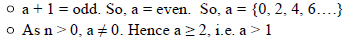Now, we don’t need to analyze if the power of other prime factors of n is greater than 1, because as we have analyzed in steps 1&2 that even if one of the prime factors of n has a power greater than 1, then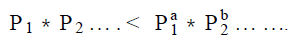However, the other prime factors of n will also have an even power greater than 1.
So, b, c …. > 1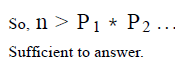Step 4 : Analyze Statement 2 independent

2. n can be expressed as a multiple of 4.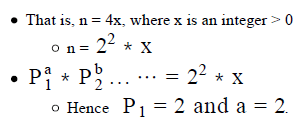• As a > 1, the answer to the question is YES

Step 5: Analyze Both Statements Together (if needed)

As we have a unique answer from steps 3 and 4, this step is not required.

QUESTION: 15

For any integer P greater than 1, P! denotes the product of all the integers from 1 to P, inclusive. If 10! Is divisible by 10080h and h
is a perfect square, what is the greatest possible value of h?

Solution:

Given:

• For a positive integer P, P! = P(P-1)(P-2) . . . * 3* 2 * 1
• 10! Is divisible by 10080*h
• 10! = 10*9*8*7*6*5*4*3*2*1
• Since 10! Is divisible by h, h is definitely non-zero (since division by 0 is not defined)
• h is a perfect square
• All perfect squares are non-negative
• Since we’ve inferred above that h is non-zero, h must be a positive integer

To Find: The greatest possible value of h

Approach:

1. We are given that 10! Is divisible by 10080*h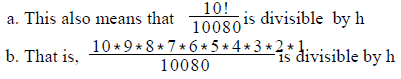2 So, we will first find the value of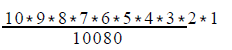3. Then, we will prime- factorize this value to determine the greatest perfect square that divides this value.

Working out: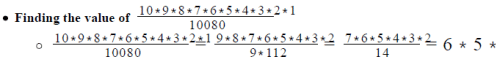• Find the greatest possible value of h
• 6*5*4*3 is divisible by perfect square h
• Writing the prime-factorized form of 6*5*4*3
•  6*5*4*3 =(3*2)*5*22*3 =23*32*5
• The greatest perfect square that divides  23*32*5 is 22 * 32
• So, the greatest possible value of h =22 * 32 = 36
Looking at the answer choices, we see that the correct answer is Option B
QUESTION: 16

How many odd positive integers divide the positive integer n completely?
(1) 16 is the highest power of 2 that divides n
(2) n has a total of 68 factors and 3 prime factors.

Solution:

Step 1 & 2: Understand Question and Draw Inference

• n is an integer > 0
• We can write: n = P1a * P2b * P3c ….where P1 , P2 , P3 ..etc. are prime numbers and a, b, c are positive integers

To Find: Number of odd positive integers divide n?

• These are the factors that do not have 2 as their prime factor.
• So, the factors which can be written in the form P1a * P2b ….where P1, P2 ... etc. are greater than 2. The number of such factors = (a+1) (b+1)….
• So, we need to find the powers of all odd prime factors of n.

Step 3 : Analyze Statement 1 independent

1. 16 is the highest power of 2 that divides n

Statement-1 tells us that the prime number 2 comes 16 times in n.
So, for n = P1a * P2b * P3c ….we know that P1 = 2 and a = 16.
However it does not tell us anything about the powers of odd prime factors of n.
Hence statement-1 is insufficient to answer the question.

Step 4 : Analyze Statement 2 independent

2. n has a total of 68 factors and 3 prime factors.
Statement-2 tells us that n has a total of 68 factors and 3 prime factors.
So, for n = P1a * P2b * P3c  we know that (a+1) (b+1) (c+1) = 68.
Now, let’s find the number of ways in which 68 can be expressed as a product of 3 integers > 1.
68 = 2 * 2 * 17.
So, (a, b, c) = (1, 1, 16). However, we do not know:
If 2 is a prime factor of n AND
Even if 2 is a prime factor of n, the power of 2 in n.
So, we cannot in any way find out the number of odd positive integers that divide n.
Hence statement-2 is insufficient to answer the question.

Step 5: Analyze Both Statements Together (if needed)

Since we do not have a unique answer from either step 3 or 4, we need to
analyze both the steps together.
Step-3 tells us that P1 = 2 and a = 16.
Step- 4 tells us that n = P1a * P2b * P3c and (a+1) (b+1) (c+1) = 68.
Combining both the steps, we can say that (b+1) (c+1) = 4 i.e. there are a total
of 4 odd factors of n.
Hence statements 1 and 2 together are sufficient to answer the question.

QUESTION: 17

For any integer x greater than 1, x! denotes the product of all integers from 1 to x, inclusive. What is the hundred-thousands
digit of 25!?

Solution:

Given:

• x is an integer > 1
• Then x! = 1*2*3………x

To Find:: Hundred-thousand digit of 25!

• 25! = 1*2*3…..25
• Hundred-thousand digit of 25! is the digit, that comes at the 6 place from
• the rightmost digit, in the value of 25!.

Approach:

1.  Let’s first try to find the number of zeroes at the end in 25!. If there are more than 5 zeroes, then the hundred-thousands digit will be 0
2. Now, 0 can occur only when a 5 is multiplied with an even number
• So, if 5 is multiplied with any multiple of 2, it will result in a zero.
3. So, we would try to find the number of 2s and 5s in 25!, i.e. the power of 2 and 5 in 25!
• Let’s take a simple example to understand how we can find this. Consider a number p = 1*2*3*4. We need to find the power of 2 in p.
• Now, powers of 2 will occur in multiples of 2. So, we should first find the multiples of 2 in p. They are 2 and 4. However all multiples of 2 will not contain only 2 . Some of them may contain higher powers of 2. For example, here 4 contains 22.
• So, when we are finding multiples of 2, we should also find the multiples of higher powers of 2 in p.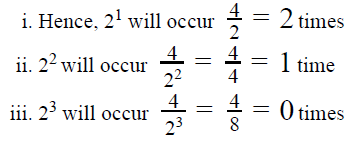• Hence, 2 will occur 2 + 1 = 3 times in p
• Using the same analogy, we will find the power of 2 and 5 in 25!
• Once we get the power of 2 and 5 in 25!, we can calculate the number of zeroes at the end of 25

Working out: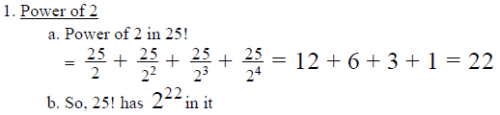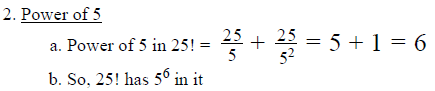3. As 25! has 222 and 56 in it, 25! will have 10 in it

• This is because 5 is the limiting factor here, as it has a lesser power than 2.

4. So, 25! will have 6 zeroes at the end. Hence, the hundred-thousands digit will be 0.

QUESTION: 18

What is the number of positive integers that divide k but do not divide k, where k is a positive integer?
(1) k2 has a total of 13 factors
(2) √k has a total of 4 factors

Solution:

Step 1 & 2: Understand Question and Draw Inference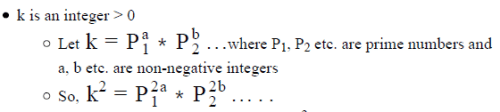To Find: number of positive integers that divide k2 but do not divide k

• Number of integers that divide k2 = factors of k2
• Now, all the integers that are a factor of k must be the factors of k2 (As k is a factor of k2 )
• So, the factors of k2 that do not divide k = Factors(k2 ) - Factors(k)
• Factors (k)= (a+1)(b+1)….
• Factors (k2 = (2a +1) *(2b +1)….
• So, we need to find the value of: (2a +1) *(2b +1)…. - (a+1)(b+1)….

Step 3 : Analyze Statement 1 independent

1. k2 has a total of 13 factors

• Number of Factors (k2 = (2a+1)(2b+1)… = 13.
• As 13 is a prime number, there is only one way to express 13 as a product of two or more positive integers, i.e. 13 * 1
• So, (2a+1)*(2b+1)… = 13* 1
• So, 2a+1 = 13 and 2b + 1= 1
• a = 6 and b = 0
• Thus k = P16 and k2 = P112
• Number of Factors (k) = 7 and Factors (k2) = 13

Hence, there are a total of 6 positive integers that divide k but do not divide k. Sufficient to answer

Step 4 : Analyze Statement 2 independent

1. √k has a total of 4 factors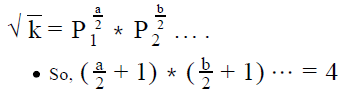• Now 4 can be expressed as a product of two or more positive integers in the following possible ways:
•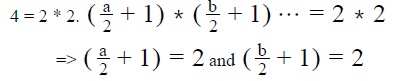•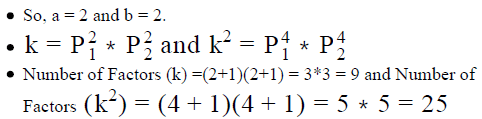• Thus, there will be 25 – 9 = 16 factors that will divide k2 but will not divide k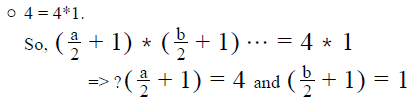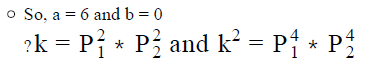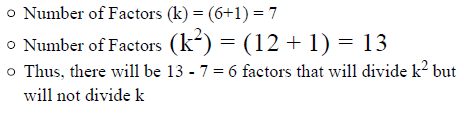As we do not have a unique answer, this statement is insufficient to answer.

Step 5: Analyze Both Statements Together (if needed)

As we have a unique answer from step-3, this step is not required

QUESTION: 19

For any integer P greater than 1, P! denotes the product of all the integers from 1 to P, inclusive. What is the greatest integer m for
which 45m is a factor of 48!?

Solution:

Given:

• For any positive integer P, P! = P(P-1)(P-2) . . . * 3* 2 * 1
• Since 48 is a positive integer, 48! = 48*47*46*45* . . . *3*2*1

To Find:  The greatest integer m for which 45m is a factor of 48!

Approach:

1. To determine the greatest possible value of m, we first need to understand when will 45 be a factor of 48!

45m = (32 *5)m = 32m *5m
So, 45m is a factor of 48! only if:
a. 32m is a factor of 48! AND
b. 5m is a factor of 48!

We need to find the greatest value of m that satisfies this constraint

2. We’ll find the greatest value of m for which 32m is a factor of 48! Let this value be x

• To find x, we’ll calculate the power of 3 in the prime-factorized expression of 48!

3. Next, we’ll find the greatest value of m for which 5m is a factor of 48! Let this value be y

• To find y, we’ll calculate the power of 5 in the prime-factorized expression of 48!

4. The greatest value of m for which 32m and 5m are both factors of 48! will be equal to the lesser value between x and y

Working out:

• Finding the value of x
• We first need to calculate the power of 3 in the prime-factorized expression for 48!
• As inferred above, 48! = 48*47*46*45* . . . *3*2*1
• The multiples of 3 in the above product are: {3, 6, 9, 12, 15, 18, 21, 24 . . . 48}
• Upon counting, we see that there are 16 multiples of 3
• Note: To ease the counting, here’s a simple trick: 3 = 3*1 and 48 = 3*16. This means, starting from 3 till 48, inclusive, there are 16 multiples of 3
• So, each of these multiples of 3 contributes at least one 3 to the primefactorized form of 48!
• So, the number of 3s we’ve counted so far = 16
• Now, note that some multiples of 3 in the list above are also multiples of 9 (= 3 ). These multiples therefore contain not one but two 3s. The number 16 calculated above considers only one 3 contributed by each multiple. So, we need to know how many multiples of 9 are there between 1 and 48 to know how many additional 3s they contribute.
• There are 5 multiples of 9 between 1 and 48.
• So, these 5 multiples contribute at least one more 3 each to the primefactorized form of 48! (in addition to the one 3 already counted when counting the multiples of 3 in the above point)
• So, the number of 3’s counted so far = 16 + 5 = 21
• Note that one multiple of 9 in the list above is also a multiple of 27 = 33. This is the number 27 itself. So, this number contributes one more 3 to the prime-factorized form of 48!
• So, the total number of 3s in the prime-factorized form of 48! = 21 + 1 = 22
• Therefore, the power of 3 in the prime-factorized form of 48! Is 22
• Hence, the maximum power of 3 which can divide 48! completely is 322

• So, for 32m to divide 48!
• The maximum value of 2m = 22
• The maximum value of m = 11
• That is, x = 11

Important Takeaway:

We can summarize the process we used above to find the power of 3 in 48!, in the following formula:

(Power of 3 in 48!)
= (Number of multiples of 3 in 48!) + (Number of multiples of 32 in 48!) + (Number of multiples of 33 in 48!)

Good so far?

Now comes the interesting point:

We can follow this same process to find the power of any prime number X in P! where P is any positive integer

The general formula to summarize the above process will be:

(Power of prime-factor X in P!)
= (Number of multiples of X in P!) + (Number of multiples of X2 in P!) + (Number of multiples of X3 in P!) + ...

• Finding the value of y
• We first need to find the power of 5 in the prime-factorized expression for 48! This time, we’ll use the formula to find this power.
• Once again, we’ll start by writing 48! = 48*47*46*45* . . . *3*2*1
• The multiples of 5 in the above product are: {5, 10, 15 . . . 45}
• Upon counting, we see that there are 9 multiples of 5

• There is only 1 multiple of 52 (that is, 25) in 48!. This multiple is number 25 itself.
• So, the power of 5 in the prime-factorized form of 48! = 9 + 1 = 10
• Therefore, the maximum power of 5 which can divide 48! completely is 510

• So, for 5m to divide 48!
• The maximum value of m = 10
• That is, y = 10

• Finding the greatest possible value of m
• We’ve determined that x = 11 and y = 10
• The lesser value out of 10 and 11 is 10
• So, the greatest value of m for which 45m is a factor of 48! Is 10 (because if m = 11 then 32m = 322 will be a factor of 48! But 5m =511 will not be a factor of 48!)

Looking at the answer choices, we see that the correct answer is Option D

QUESTION: 20

If z is the product of integers from 1 to 51, inclusive, what is the greatest value of k+ m, where k and m are integers greater than 1,
for which 45k * 32m is a factor of z?

Solution:

Given:

• z = 1*2*3…………..*51
• k, m are integers > 1

To Find: Greatest value of k + m , for which 45 * 32 is a factor of z ?

Approach:

1. To find the greatest possible value of k + m, we need to find the greatest possible value of k and the greatest possible value of m under the given constraint.
2. The Constraint: 45 * 32 is a factor of z.
• Since 45 * 32 is a factor of z, we can write: z = (45k * 32m ) * x, where quotient x is an integer > 0
3. For z = 45k * 32m * x, z should contain the prime factors of 45 raised to power k and prime-factorized expression of 32 raised to power m.
1. So, we will first find the value of prime factorized expression of 45 and 32 raised to their respective powers.
2. Since the powers of 45 and 32 are k and m respectively, we’ll get the powers of prime factors of 45 and 32 too in terms of k and m
4. Now, we’ll find the powers of these prime factors in z. Equating these powers to the respective powers obtained in Step 3b above
5. We will find the maximum value of k and m.

Working out: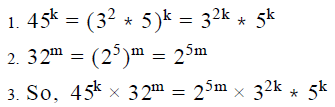4. Next, we need to find the number of times, 2, 3 and 5 occur in z = 1*2*3…….51
a. Let’s take a simple example to understand how we can find this. Consider a number p = 1*2*3*4. We need to find the power of 2 in p.

b. Now, powers of 2 will occur in multiples of 2. So, we should first find the multiples of 2 in p. They are 2 and 4. However all multiples of 2 will not contain only 21 . Some of them may contain higher powers of 2. For example, here 4 contain 22 .
c. So, when we are finding multiples of 2, we should also find the multiples of higher powers of 2 in p.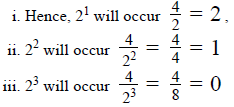d. Hence, 2 will occur 2 + 1 = 3 times in p.
5. Using the same analogy, let’s find out the powers of 2, 3 and 5 in z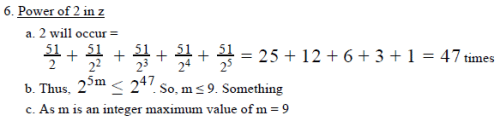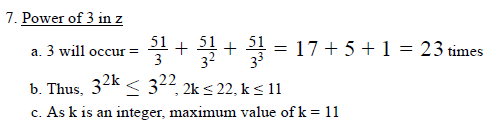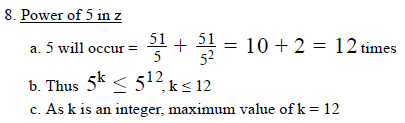9. Using points (6.c) and (7.c), we can say that maximum value of k = 11

• This is because for 45k , we need both 32k and to  5occur the same number of times. As 32k can occur 11 times and 5can occur 12 times, the maximum power of 45k can be 11. Hence maximum value of k = 11

10. Hence, maximum (k + m ) = 11 + 9 = 20Use Code STAYHOME200 and get INR 200 additional OFF Use Coupon Code